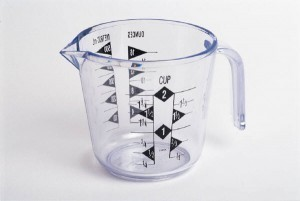# Volume Measurements

Whether you are cooking or storing material, a volume measurementguide can remove a lot of the difficulties when dealing with different figures.

### Volume Conversion Guide: Measurements in Cooking

1 tablespoon is equal to 3 teaspoons and 1 fluid ounce is equivalent to 2 tablespoons. 8 ounces is equivalent to a cup, while 2 cups is the same as a pint. 2 pints is equal to a quart and four quarts make up a gallon. ¼ teaspoon is the same as 1.25 milliliters.

1/2 teaspoon is the same as 2.5 milliliters. A single teaspoon is the same as 5 milliliters. 15 milliliters is equal to 1 tablespoon. 30 milliliters is equal to 1 fluid ounce. 1/3 cup is the same as 60 milliliters while 78 milliliters is equal to 1/3 cup. ½ cup is the same as 118.2 milliliters. A cup is equivalent to 236.5 milliliters.

### Volume Measurement Guide: Pints, Quarts, Gallons and Liters

1 pint (U.S.) is equal to 473 milliliters. A pint is also the same as 28.875 cubic Inches, 16 ounces and 0.4732 liters. 1 quart (US) is equal to 946 milliliters, 4 cups and 32 ounces. 1 US gallon is equivalent to 128 ounces. 1 gallon (U.S.) is the same as 3.79 liters or 3785 cubic centimeters. A US gallon is also equal to 4 quarts and 8 pints.

1 liter is equal to 1000 cubic centimeters, 1.057 quarts and 1.76 pints. The gallon is also the same as 35.2 fluid ounces, 1000 milliliters and 0.264 gallons.

### Volume Measurement of Objects

For regularly shaped objects like squares and rectangles, measure the length, width and height of the object. When you have the figures, multiply them (L x W x H). The answer is the volume’s object. For irregularly shaped solid objects, you must use a graduated cylinder, water and overflow can.

A graduated cylinder is a lab tool designed to measure liquid volume. Its size ranges from 10 milliliters to 2 liters. The overflow can is where the irregularly shaped object will be put. Their downspouts can be angled or straight.

To get the volume measurement, pour water in the overflow can. Allow the excess water to go to the downspout. Place the graduated cylinder below the downspout. Place the irregularly shaped object in it. Let the water run to the graduated cylinder. Now read the water amount that was moved from the can to the cylinder. This is the object’s volume.

### Old Measurements and their Quart Equivalents

In biblical times, a bath can be equal to 0.04166667 quarts while a hin is equal to 0.25. A log is 3 quarts. The old Roman quart is equal to 6.958477 quarts today. The Old French minot is equal to 0.02783391 quarts while the Old Russian vodka bottle is equivalent to 1.538785 quarts.

The vine bottle is equal to 1.231028 quarts while the chetvert (quart) is 0.3077571 quarts today.

There are other volume measurements used during ancient times. However, most of them are no longer used except for historical research.